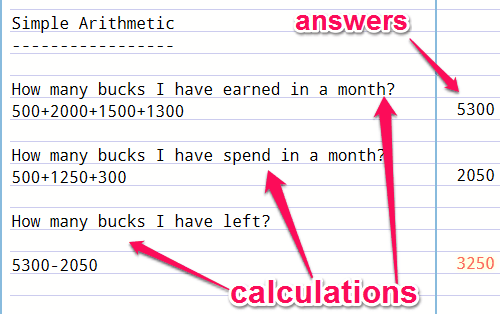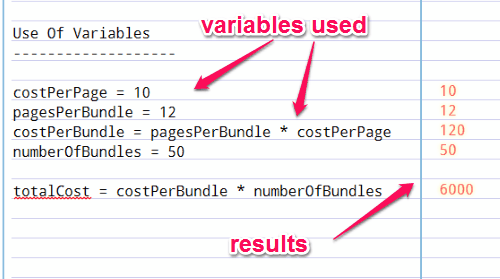Editor Ratings:
User Ratings:
[Total: 0 Average: 0]

Notepad Calculator is a free online notepad that supports real-time arithmetic calculations. This app is very useful for doing quick calculations along with taking notes. You can do simple arithmetic or use variables and get instant result side by side. It enables you to do calculations over multiple lines and lets you write details alongside about the calculation so that the calculation makes sense. Unlike, the other online notepads, this website is a combination of a notepad and a calculator which lets you perform simple math operations with ease. Sometimes, you might find it difficult to use the default system calculator as you have to remember all the previous calculations. But this website solves this problem and lets you do calculations in multiple lines. It comes with a single page web application which allows you to mix text and calculations together and also supports the use of variables for calculations. The interface is very simple and you can learn to use the website by simply playing around with it.

The following screenshot shows the interface of this online notepad with calculations.All of your calculations are stored within the browser’s internal storage (cache memory), which means next time you open the website, you will be able to see your previous calculations.

Note: As soon as you clear the browser cookies, all of your previous calculations and notes will be lost.

## How to Use “Notepad Calculator” to do Calculations:

Using this website is a very easy task and doesn’t require any Rocket Science. So, let us see the steps of using this website for simple and variable calculations.

### Do simple calculations:

You can do simple arithmetic calculations by simply typing the numbers and operations in the web application. For example, you want to note down some calculations about your earning then write details about the calculation and go ahead to start calculating. The website provides the calculation results side by side in real-time. The following screenshot shows how to do simple arithmetic calculations.### Use variables for calculation:

The best thing about this website is that it supports the use of variables for calculations. In addition to simple arithmetic calculations, you can go for some variable calculations too. Let’s say, you want to calculate the total cost of printing a page, the total number of pages, etc. The following screenshot shows how to use variables for calculations.## My Final Verdict:

Notepad Calculator is a very useful online notepad application which lets you do quick calculations within the notepad. It supports simple arithmetic calculations as well as the use of variables. If you want some quick arithmetic calculations then using this website instead of the default system calculator would be the best way to do so.

Try the website from the link here.